## Saturday, 9 August 2014

### CHAPTER 7 - Worked Out Examples 2

 Example: 13
Find the angle of intersection of the two planes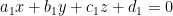${a_1}x + {b_1}y + {c_1}z + {d_1} = 0$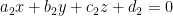${a_2}x + {b_2}y + {c_2}z + {d_2} = 0$
 Solution: 13
From the equations of the planes, it is evident that the following vectors are to these planes arespectively: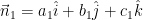${\vec n_1} = {a_1}\hat i + {b_1}\hat j + {c_1}\hat k$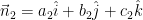${\vec n_2} = {a_2}\hat i + {b_2}\hat j + {c_2}\hat k$
Since the acute angle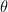$\theta$ between the two planes will be the acute angle between their normals, we have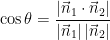$\cos \theta = \dfrac{{\left| {{{\vec n}_1} \cdot {{\vec n}_2}} \right|}}{{\left| {{{\vec n}_1}} \right|\left| {{{\vec n}_2}} \right|}}$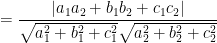$= \dfrac{{\left| {{a_1}{a_2} + {b_1}{b_2} + {c_1}{c_2}} \right|}}{{\sqrt {a_1^2 + b_1^2 + c_1^2} \sqrt {a_2^2 + b_2^2 + c_2^2} }}$
Incidentally, we can now derive the conditions for these planes to be parallel or perpendicular.
Planes are parallel if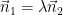${\vec n_1} = \lambda {\vec n_2}$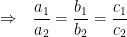$\Rightarrow\,\,\,\, \dfrac{{{a_1}}}{{{a_2}}} = \dfrac{{{b_1}}}{{{b_2}}} = \dfrac{{{c_1}}}{{{c_2}}}$
Plames are perpendicular if${\vec n_1} \times {\vec n_2} = 0$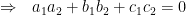$\Rightarrow\,\,\,\, {a_1}{a_2} + {b_1}{b_2} + {c_1}{c_2} = 0$
It should be obvious that for two parallel planes, their equations can be written so that they differ only in the constant term. Thus, any plane parallel to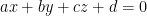$ax + by + cz + d = 0$ can be written as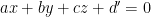$ax + by + cz + d' = 0$ where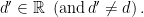$d' \in \mathbb{R}\,\,\left( {{\rm{and }}\,d' \ne d} \right).$
 Example: 14
 (a) Find the distance of the point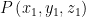$P\left( {{x_1},{y_1},{z_1}} \right)$ from the plane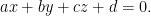$ax + by + cz + d = 0.$ (b) Find the distance beween the two parallel lines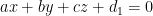$ax + by + cz + {d_1} = 0$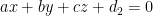$ax + by + cz + {d_2} = 0$
 Solution: 14-(a)
The distance$l$ of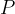$P$ from the given plane will obviously be measured along the normal to the plane passing through$P$:We write the equation of the plane as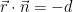$\vec r \cdot \vec n = - d$
where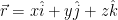$\vec r = x\hat i + y\hat j + z\hat k$ is any point on the plane and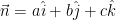$\vec n = a\hat i + b\hat j + c\hat k$ is the normal to the plane.
Let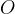$O$ be the origin. Since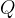$Q$ lies on the plane, its position vector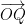$\overrightarrow {OQ}$ must satisfy the equation of the plane. But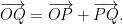$\overrightarrow {OQ} = \overrightarrow {OP} + \overrightarrow {PQ} .$ Thus,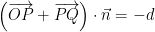$\left( {\overrightarrow {OP} + \overrightarrow {PQ} } \right) \cdot \vec n = - d$
Note that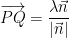$\overrightarrow {PQ} = \dfrac{{\lambda \vec n}}{{\left| {\vec n} \right|}}$ where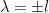$\lambda = \pm l$ (which sign to take depends on which direction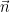$\vec n$ points in).
Thus,$\left( {\overrightarrow {OP} + \dfrac{{\lambda \vec n}}{{\left| {\vec n} \right|}}} \right) \cdot \vec n = - d$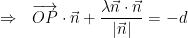$\Rightarrow \,\,\,\, \overrightarrow {OP} \cdot \vec n + \dfrac{{\lambda \vec n \cdot \vec n}}{{\left| {\vec n} \right|}} = - d$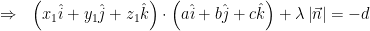$\Rightarrow \,\,\,\, \left( {{x_1}\hat i + {y_1}\hat j + {z_1}\hat k} \right) \cdot \left( {a\hat i + b\hat j + c\hat k} \right) + \lambda \left| {\vec n} \right| = - d$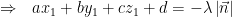$\Rightarrow \,\,\,\, a{x_1} + b{y_1} + c{z_1} + d = - \lambda \left| {\vec n} \right|$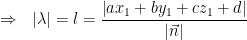$\Rightarrow \,\,\,\, \left| \lambda \right| = l = \dfrac{{\left| {a{x_1} + b{y_1} + c{z_1} + d} \right|}}{{\left| {\vec n} \right|}}$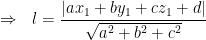$\Rightarrow \,\,\,\, l = \dfrac{{\left| {a{x_1} + b{y_1} + c{z_1} + d} \right|}}{{\sqrt {{a^2} + {b^2} + {c^2}} }}$
 Solution: 14-(b)
Assume any point$P\left( {{x_1},{y_1},{z_1}} \right)$ on the first plane. We have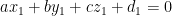$a{x_1} + b{y_1} + c{z_1} + {d_1} = 0$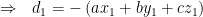$\Rightarrow \,\,\,\, {d_1} = - \left( {a{x_1} + b{y_1} + c{z_1}} \right)$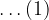$\ldots(1)$
The distance of$P$ from the second plane, say$l$, can be evaluated as described in part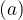$(a)$ above :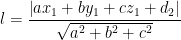$l = \dfrac{{\left| {a{x_1} + b{y_1} + c{z_1} + {d_2}} \right|}}{{\sqrt {{a^2} + {b^2} + {c^2}} }}$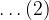$\ldots(2)$
Using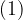$(1)$ in$(2)$ we have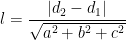$l = \dfrac{{\left| {{d_2} - {d_1}} \right|}}{{\sqrt {{a^2} + {b^2} + {c^2}} }}$
This is the required distance between the two planes